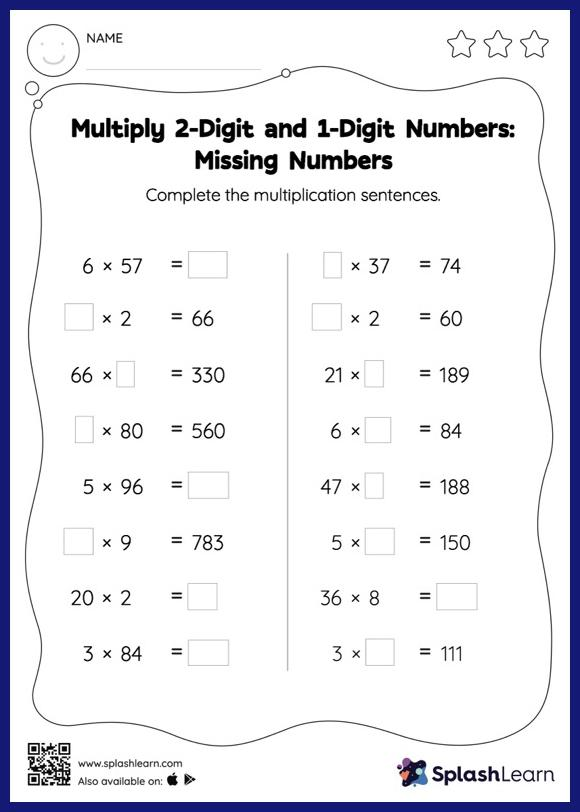# Multiply 2-Digit and 1-Digit Numbers: Missing Numbers Worksheet

Home > Multiply 2-Digit and 1-Digit Numbers: Missing NumbersWhen multiplying a multi-digit number by a one-digit number, students multiply one digit at a time. They find the missing number in the multiply 2-digit and 1-digit numbers worksheet by using their knowledge of basic multiplication facts. This worksheet is about practicing with the horizontal format in which numbers are written side by side. To develop flexibility with numbers and operations, students need to have enough practice in this format and not just rely on the vertical/column method.